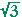Topics in

P R E C A L C U L U S

11

# COMPLETING THE SQUARE

IF WE TRY TO SOLVE this quadratic equation by factoring

x2 + 6x + 2  =  0

we cannot.  Therefore, we use a method called completing the square. That means to make the quadratic into a perfect square trinomial, i.e. the form

a2 + 2ab + b2  =  (a + b)2.

This method is valid only when 1 is the coefficient of x2.

1)  Transpose the constant term to the right:

x2 + 6x  = −2

2)  Add a square number to both sides: Add the square of half the coefficient of x.  In this case, add the square of 3:

x2 + 6x + 9  =  −2 + 9

The left-hand side is now the perfect square of  (x + 3).

(x + 3)2  =  7.

That equation has the form

 a2 = b, which implies a = ±. Therefore, x + 3 = ±x = −3 ±.

The solutions to

x2 + 6x + 2  =  0

are the conjugate pair,

−3 +,  −3 −.

We can check this.  The sum of those roots is −6, which is the negative of the coefficient of x.  And the product of the roots is

(−3)2 − ()2  =  9 − 7  =  2,

which is the constant term.  Thus both conditions on the roots are satisfied.  Those are the two roots of the quadratic.

Problem.   Solve this quadratic equation by completing the square.

x2 − 2x − 2 = 0

To see the solution, pass your mouse over the colored area.
To cover the solution again, click "Refresh" ("Reload").

 x2 − 2x = 2 x2 − 2x + 1 = 2 + 1 (x − 1)2 = 3 x − 1 = ±x = 1 ±See Lesson 37 of Algebra, Problems 6 and 7.

Before considering the quadratic formula, note that half of any

 number b is b2 .   Half of  5 is 52 .   Half of pq is p2q .

(Lesson 27 of Arithmetic, Question 4.)

Theorem.   If

ax2 + bx + c = 0,

Theorem.   thenTo prove this, we will complete the square.  But to do that, the coefficient of x2 must be 1.  Therefore, we will divide both sides of the original equation by a:Next Topic:  Synthetic division by xa

Please make a donation to keep TheMathPage online.
Even \$1 will help.